# Version information¶

:

from datetime import date
print("Running date:", date.today().strftime("%B %d, %Y"))
import pyleecan
print("Pyleecan version:" + pyleecan.__version__)
import SciDataTool
print("SciDataTool version:" + SciDataTool.__version__)

Running date: April 29, 2021
Pyleecan version:1.2.1
SciDataTool version:1.3.1


# How to define a machine¶

This tutorial shows the different ways to define electrical machine. To do so, it presents the definition of the Toyota Prius 2004 interior magnet with distributed winding .

The notebook related to this tutorial is available on GitHub.

## Type of machines Pyleecan can model¶

Pyleecan handles the geometrical modelling of main 2D radial flux machines such as:
- surface or interior permanent magnet machines (SPMSM, IPMSM)
- synchronous reluctance machines (SynRM)
- squirrel-cage induction machines and doubly-fed induction machines (SCIM, DFIM)
- would rotor synchronous machines and salient pole synchronous machines (WSRM)
- switched reluctance machines (SRM)

The architecture of Pyleecan also enables to define other kinds of machines (with more than two laminations for instance). More information in our ICEM 2020 pyblication 

Every machine can be defined by using the Graphical User Interface or directly in Python script.

## Defining machine with Pyleecan GUI¶

The GUI is the easiest way to define machine in Pyleecan. Its purpose is to create or load a machine and save it in JSON format to be loaded in a python script. The interface enables to define step by step in a user-friendly way every characteristics of the machine such as:
- topology
- dimensions
- materials
- winding

Each parameter is explained by a tooltip and the machine can be previewed at each stage of the design.

## Start the GUI¶

The GUI can be started by running the following command in a notebook:

# Start Pyleecan GUI from the Jupyter Notebook
%run -m pyleecan


To use it on Anaconda you may need to create the system variable:

QT_QPA_PLATFORM_PLUGIN_PATH : path:nbsphinx-math:to\anaconda3\Lib\site-packages:nbsphinx-math:PySide2\plugins\platforms

The GUI can also be launched in a terminal by calling one of the following commands in a terminal:

Path/to/python.exe -m pyleecan
Path/to/python3.exe -m pyleecan


## load a machine¶

Once the machine defined in the GUI it can be loaded with the following commands:

:

%matplotlib notebook

# Load the machine
from os.path import join
from pyleecan.Functions.load import load
from pyleecan.definitions import DATA_DIR

IPMSM_A = load(join(DATA_DIR, "Machine", "Toyota_Prius.json"))
IPMSM_A.plot()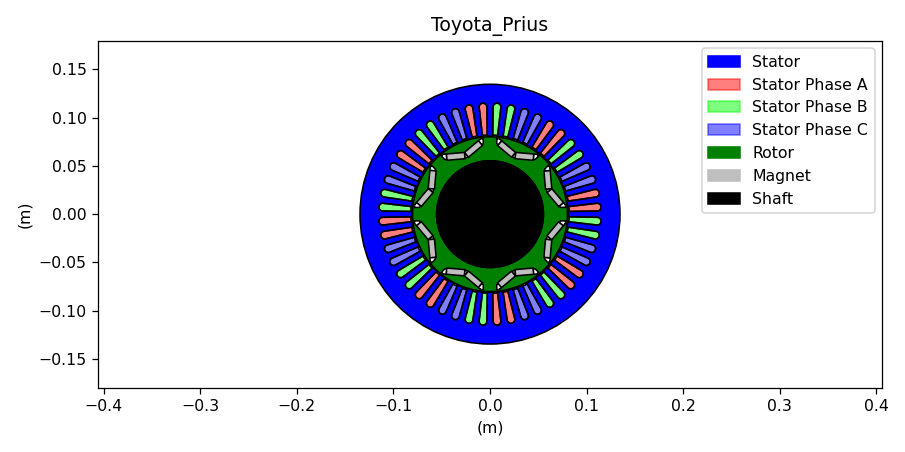c:\python38\lib\site-packages\pyleecan\Methods\Machine\Machine\plot.py:123: MatplotlibDeprecationWarning:
The set_window_title function was deprecated in Matplotlib 3.4 and will be removed two minor releases later. Use manager.set_window_title or GUI-specific methods instead.
fig.canvas.set_window_title(self.name + " plot machine")


## Defining Machine in scripting mode¶

Pyleecan also enables to define the machine in scripting mode, using different classes. Each class is defined from a csv file in the folder pyleecan/Generator/ClasseRef and the documentation of every class is available on the dedicated webpage. The following image shows the machine classes organization :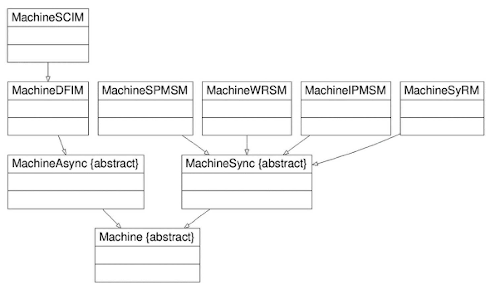Every rotor and stator can be created with the Lamination class or one of its daughters.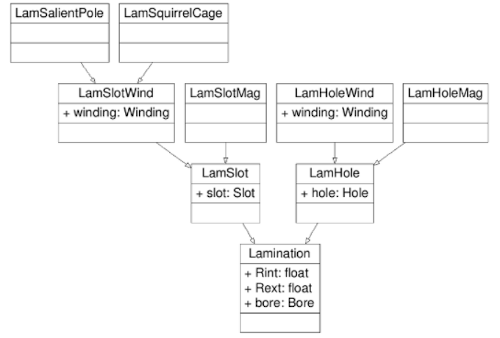The scripting enables to define some complex and exotic machine that can’t be defined in the GUI such as this one:

:

from pyleecan.Classes.MachineUD import MachineUD
from pyleecan.Classes.LamSlotWind import LamSlotWind
from pyleecan.Classes.LamSlot import LamSlot
from pyleecan.Classes.WindingCW2LT import WindingCW2LT
from pyleecan.Classes.SlotW10 import SlotW10
from pyleecan.Classes.SlotW22 import SlotW22
from numpy import pi

machine = MachineUD(name="4 laminations machine")

# Main geometry parameter
Rext = 170e-3  # Exterior radius of outter lamination
W1 = 30e-3  # Width of first lamination
A1 = 2.5e-3  # Width of the first airgap
W2 = 20e-3
A2 = 10e-3
W3 = 20e-3
A3 = 2.5e-3
W4 = 60e-3

# Outer stator
lam1 = LamSlotWind(Rext=Rext, Rint=Rext - W1, is_internal=False, is_stator=True)
lam1.slot = SlotW22(
Zs=12, W0=2 * pi / 12 * 0.75, W2=2 * pi / 12 * 0.75, H0=0, H2=W1 * 0.65
)
lam1.winding = WindingCW2LT(qs=3, p=3)
# Outer rotor
lam2 = LamSlot(
Rext=lam1.Rint - A1, Rint=lam1.Rint - A1 - W2, is_internal=True, is_stator=False
)
lam2.slot = SlotW10(Zs=22, W0=25e-3, W1=25e-3, W2=15e-3, H0=0, H1=0, H2=W2 * 0.75)
# Inner rotor
lam3 = LamSlot(
Rext=lam2.Rint - A2,
Rint=lam2.Rint - A2 - W3,
is_internal=False,
is_stator=False,
)
lam3.slot = SlotW10(
Zs=22, W0=17.5e-3, W1=17.5e-3, W2=12.5e-3, H0=0, H1=0, H2=W3 * 0.75
)
# Inner stator
lam4 = LamSlotWind(
Rext=lam3.Rint - A3, Rint=lam3.Rint - A3 - W4, is_internal=True, is_stator=True
)
lam4.slot = SlotW10(Zs=12, W0=25e-3, W1=25e-3, W2=1e-3, H0=0, H1=0, H2=W4 * 0.75)
lam4.winding = WindingCW2LT(qs=3, p=3)
# Machine definition
machine.lam_list = [lam1, lam2, lam3, lam4]

# Plot, check and save
machine.plot()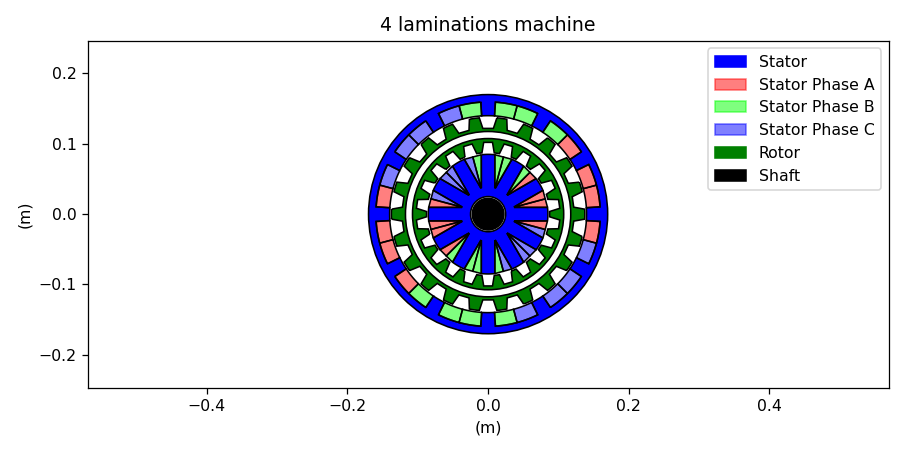## Stator definition¶

To define the stator, we initialize a LamSlotWind object with the different parameters. In pyleecan, all the parameters must be set in SI units.

:

from pyleecan.Classes.LamSlotWind import LamSlotWind
mm = 1e-3  # Millimeter

# Lamination setup
stator = LamSlotWind(
Rint=80.95 * mm, # internal radius [m]
Rext=134.62 * mm, # external radius [m]
L1=83.82 * mm, # Lamination stack active length [m] without radial ventilation airducts
# but including insulation layers between lamination sheets
Nrvd=0, # Number of radial air ventilation duct
Kf1=0.95, # Lamination stacking / packing factor
is_internal=False,
is_stator=True,
)


Then we add 48 slots using SlotW11 which is one of the 25 Slot classes:

:

from pyleecan.Classes.SlotW11 import SlotW11

# Slot setup
stator.slot = SlotW11(
Zs=48, # Slot number
H0=1.0 * mm,  # Slot isthmus height
H1=0, # Height
H2=33.3 * mm, # Slot height below wedge
W0=1.93 * mm,  # Slot isthmus width
W1=5 * mm, # Slot top width
W2=8 * mm, # Slot bottom width
R1=4 * mm # Slot bottom radius
)


As for the slot, we can define the winding and its conductor with WindingDW1L and CondType11. The conventions for winding are further explained on pyleecan website

:

from pyleecan.Classes.WindingDW1L import WindingDW1L
from pyleecan.Classes.CondType11 import CondType11
# Winding setup
stator.winding = WindingDW1L(
qs=3,  # number of phases
Lewout=0,  # staight length of conductor outside lamination before EW-bend
p=4,  # number of pole pairs
Ntcoil=9,  # number of turns per coil
Npcpp=1,  # number of parallel circuits per phase
Nslot_shift_wind=0,  # 0 not to change the stator winding connection matrix built by pyleecan number
# of slots to shift the coils obtained with pyleecan winding algorithm
# (a, b, c becomes b, c, a with Nslot_shift_wind1=1)
is_reverse_wind=False # True to reverse the default winding algorithm along the airgap
# (c, b, a instead of a, b, c along the trigonometric direction)
)

# Conductor setup
stator.winding.conductor = CondType11(
Nwppc_tan=1, # stator winding number of preformed wires (strands)
# in parallel per coil along tangential (horizontal) direction
Nwppc_rad=1, # stator winding number of preformed wires (strands)
# in parallel per coil along radial (vertical) direction
Wwire=0.000912, #  single wire width without insulation [m]
Hwire=2e-3, # single wire height without insulation [m]
Wins_wire=1e-6, # winding strand insulation thickness [m]
type_winding_shape=0, # type of winding shape for end winding length calculation
# 0 for hairpin windings
# 1 for normal windings
)


## Rotor definition¶

For this example, we use the LamHole class to define the rotor as a lamination with holes to contain magnet.

In the same way as for the stator, we start by defining the lamination:

:

from pyleecan.Classes.LamHole import LamHole

# Rotor setup
rotor = LamHole(
Rint=55.32 * mm, # Internal radius
Rext=80.2 * mm, # external radius
is_internal=True,
is_stator=False,
L1=stator.L1 # Lamination stack active length [m]
# without radial ventilation airducts but including insulation layers between lamination sheets
)


After that, we can add holes with magnets to the rotor using the class HoleM50:

:

from pyleecan.Classes.HoleM50 import HoleM50
rotor.hole = list()
rotor.hole.append(
HoleM50(
Zh=8, # Number of Hole around the circumference
W0=42.0 * mm,  # Slot opening
W1=0, # Tooth width (at V bottom)
W2=0, # Distance Magnet to bottom of the V
W3=14.0 * mm, # Tooth width (at V top)
W4=18.9 * mm, # Magnet Width
H0=10.96 * mm, # Slot Depth
H1=1.5 * mm, # Distance from the lamination Bore
H2=1 * mm, # Additional depth for the magnet
H3=6.5 * mm, # Magnet Height
H4=0, # Slot top height
)
)


The holes are defined as a list to enable to create several layers of holes and/or to combine different kinds of holes

## Create a shaft and a frame¶

The classes Shaft and Frame enable to add a shaft and a frame to the machine. For this example there is no frame:

:

from pyleecan.Classes.Shaft import Shaft
from pyleecan.Classes.Frame import Frame

# Set shaft
shaft = Shaft(Drsh=rotor.Rint * 2, # Diamater of the rotor shaft [m]
# used to estimate bearing diameter for friction losses
Lshaft=1.2 # length of the rotor shaft [m]
)
frame = None


## Set materials and magnets¶

Every Pyleecan object can be saved in JSON using the method save and can be loaded with the load function. In this example, the materials M400_50A and Copper1 are loaded and set in the corresponding properties.

:

# Loading Materials
M400_50A = load(join(DATA_DIR, "Material", "M400-50A.json"))
Copper1 = load(join(DATA_DIR, "Material", "Copper1.json"))

# Set Materials
stator.mat_type = M400_50A  # Stator Lamination material
rotor.mat_type = M400_50A  # Rotor Lamination material
stator.winding.conductor.cond_mat = Copper1  # Stator winding conductor material


A material can also be defined in scripting as any other Pyleecan object. The material Magnet_prius is created with the classes Material and MatMagnetics.

:

from pyleecan.Classes.Material import Material
from pyleecan.Classes.MatMagnetics import MatMagnetics

# Defining magnets
Magnet_prius = Material(name="Magnet_prius")

# Definition of the magnetic properties of the material
Magnet_prius.mag = MatMagnetics(
mur_lin = 1.05, # Relative magnetic permeability
Hc = 902181.163126629, # Coercitivity field [A/m]
alpha_Br = -0.001, # temperature coefficient for remanent flux density /°C compared to 20°C
Brm20 = 1.24, # magnet remanence induction at 20°C [T]
Wlam = 0, # lamination sheet width without insulation [m] (0 == not laminated)
)

# Definition of the electric properties of the material
Magnet_prius.elec.rho = 1.6e-06 # Resistivity at 20°C

# Definition of the structural properties of the material
Magnet_prius.struct.rho = 7500.0 # mass per unit volume [kg/m3]


The magnet materials are set with the “magnet_X” property. Pyleecan enables to define different magnetization or material for each magnets of the holes. Here both magnets are defined identical.

:

# Set magnets in the rotor hole
rotor.hole.magnet_0.mat_type = Magnet_prius
rotor.hole.magnet_1.mat_type = Magnet_prius
rotor.hole.magnet_0.type_magnetization = 1
rotor.hole.magnet_1.type_magnetization = 1


## Create, save and plot the machine¶

Finally, the Machine object can be created with MachineIPMSM and saved using the save method.

:

from pyleecan.Classes.MachineIPMSM import MachineIPMSM

%matplotlib notebook
IPMSM_Prius_2004 = MachineIPMSM(
name="Toyota Prius 2004",
stator=stator,
rotor=rotor,
shaft=shaft,
frame=frame  # None
)
IPMSM_Prius_2004.save('IPMSM_Toyota_Prius_2004.json')

im=IPMSM_Prius_2004.plot()

[17:38:20] Saving in IPMSM_Toyota_Prius_2004.json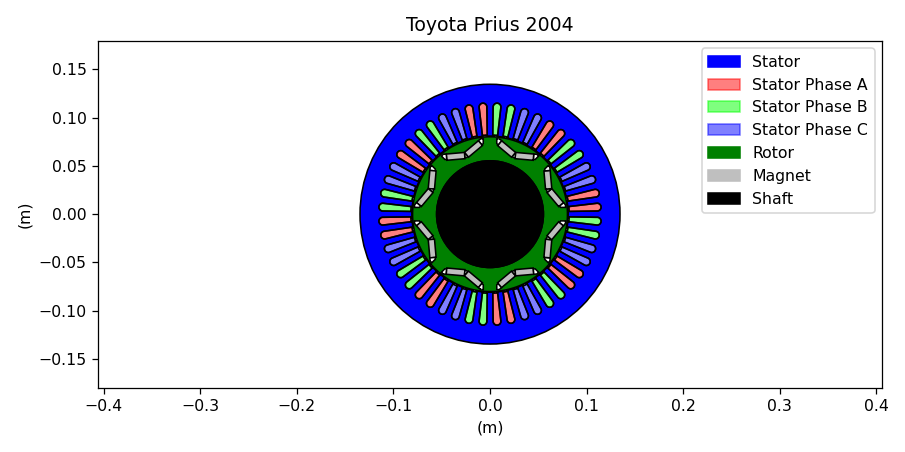Note that Pyleecan also handles ventilation duct thanks to the classes :

 Z. Yang, M. Krishnamurthy and I. P. Brown, “Electromagnetic and vibrational characteristic of IPM over full torque-speed range”, 2013 International Electric Machines & Drives Conference, Chicago, IL, 2013, pp. 295-302.

 P. Bonneel, J. Le Besnerais, E. Devillers, C. Marinel, and R. Pile, “Design Optimization of Innovative Electrical Machines Topologies Based on Pyleecan Opensource Object-Oriented Software,” in 24th International Conference on Electrical Machines (ICEM), 2020.

[ ]: